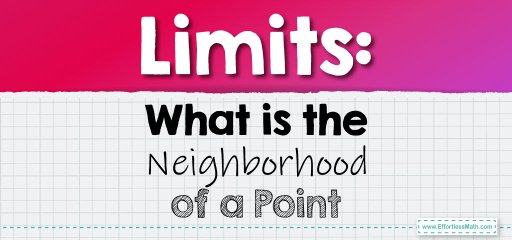# Limits: What is the Neighborhood of a Point

The concept of a neighborhood in the context of limits refers to an interval of values surrounding a specific point. A neighborhood can be thought of as a "small interval" around a particular point on the real number line.## Let’s break down the concept step by step:

### 1. Definition of a Neighborhood:

Given a point $$a$$ on the real number line and a positive number $$\epsilon$$ (no matter how small), the ε-neighborhood of $$a$$ is the set of all numbers $$x$$ such that the distance between $$x$$ and $$a$$ is less than $$\epsilon$$.

Mathematically, the ε-neighborhood of $$a$$ is:
$(a – \epsilon, a + \epsilon)$
This is an open interval, which means it doesn’t include the endpoints.

### 2. Visualizing a Neighborhood:

Imagine the real number line. The point $$a$$ is somewhere on this line. Now, you go $$\epsilon$$ units to the left and $$\epsilon$$ units to the right. The interval between these two points, excluding the points themselves, is the ε-neighborhood of $$a$$.

### 3. Connection to Limits:

The concept of a neighborhood is closely tied to the idea of a limit. When we say:
$$\lim_{x \to a} f(x) = L$$
It means that for every positive $$\epsilon$$, no matter how small, there exists a $$\delta$$ such that if $$x$$ is within the $$\delta$$-neighborhood of $$a$$ (excluding $$a$$ itself), then $$f(x)$$ is within the $$\epsilon$$-neighborhood of $$L$$.

This is a way to formalize the idea that as $$x$$ gets close to $$a$$, $$f(x)$$ gets close to $$L$$.

### 4. ε-δ Definition of a Limit:

The formal definition of a limit, using the concept of neighborhoods, is:

For every $$\epsilon > 0$$, there exists a $$\delta > 0$$ such that if $$0 < |x – a| < \delta$$ (which means $$x$$ is in the $$\delta$$-neighborhood of $$a$$ but not equal to $$a$$), then $$|f(x) – L| < \epsilon$$ (which means $$f(x)$$ is in the $$\epsilon$$-neighborhood of $$L$$).

### 5. Practice and Application:

Understanding the concept of neighborhoods and the ε-δ definition is a critical step in grasping the rigorous definition of limits. It’s common to work through multiple examples and exercises to get comfortable with the ε-δ approach.

### Recap:

To visualize a neighborhood:

1. Pick a point $$a$$ on the number line.
2. For a given $$\epsilon$$, mark the points $$a-\epsilon$$ and $$a+\epsilon$$.
3. The open interval between these two points is the ε-neighborhood of $$a$$.

The ε-δ definition of limits uses these neighborhoods to rigorously define the idea of “approaching” in calculus.

### What people say about "Limits: What is the Neighborhood of a Point - Effortless Math: We Help Students Learn to LOVE Mathematics"?

No one replied yet.

X
30% OFF

Limited time only!

Save Over 30%

SAVE $5 It was$16.99 now it is \$11.99